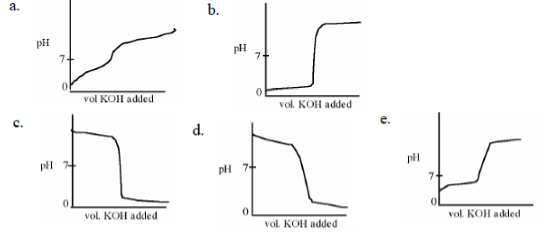Chemistry Practice Problems Acid and Base Titration Curves Practice Problems Solution: Which titration curve could describe the titration...

🤓 Based on our data, we think this question is relevant for Professor Villalta Cerdas' class at SHSU.

# Solution: Which titration curve could describe the titration of a solution of HCl by addition of a solution of KOH?

###### Problem

Which titration curve could describe the titration of a solution of HCl by addition of a solution of KOH?Acid and Base Titration Curves

Acid and Base Titration Curves

#### Q. How can you experimentally determine the pKa of acetic acid?a. Determine the pH of the solution 1/4 of the way to the end-point on the pH titration cu...

Solved • Tue Mar 31 2020 02:00:08 GMT-0400 (EDT)

Acid and Base Titration Curves

#### Q. What is the most likely identity of the species undergoing titration?a. hydrobromic acid (HBr); Ka = 1.08 x 109b. acetic acid (CH3CO2H); Ka = 1.8 x 10...

Solved • Tue Mar 31 2020 00:11:41 GMT-0400 (EDT)

Acid and Base Titration Curves

#### Q. For the pH titration below, which of the following statements is/are correct?I The titrand is a weak base that can accept two protons.II Points C and ...

Solved • Mon Feb 17 2020 14:54:02 GMT-0500 (EST)

Acid and Base Titration Curves

#### Q. Which titration curve could describe the titration of a solution of HCl by addition of a solution KOH?

Solved • Tue Feb 02 2016 13:42:42 GMT-0500 (EST)